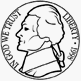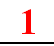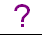Skill 8D Money Equivalents Taking money to the next step.  Here we want to see if your student knows how to make equal amounts from different coins.  The first one is done for you:  5 cents can be represented by either one nickel or five pennies.  The student selects the number from below each coin To the left of each row of coins shows a written amount of money. How many of each coin is needed to equal the amount of money shown?  Let's do the first one together.  5 cents can be shown by either one nickel or 5 pennies.  We do not want to add the coins, we only want to figure out how many of each coin equals the amount shown. You try the second row. How many of each coin is needed to show 10 cents?  Keep going until you answer all amounts. 5¢or10¢oror25¢oror50¢oror